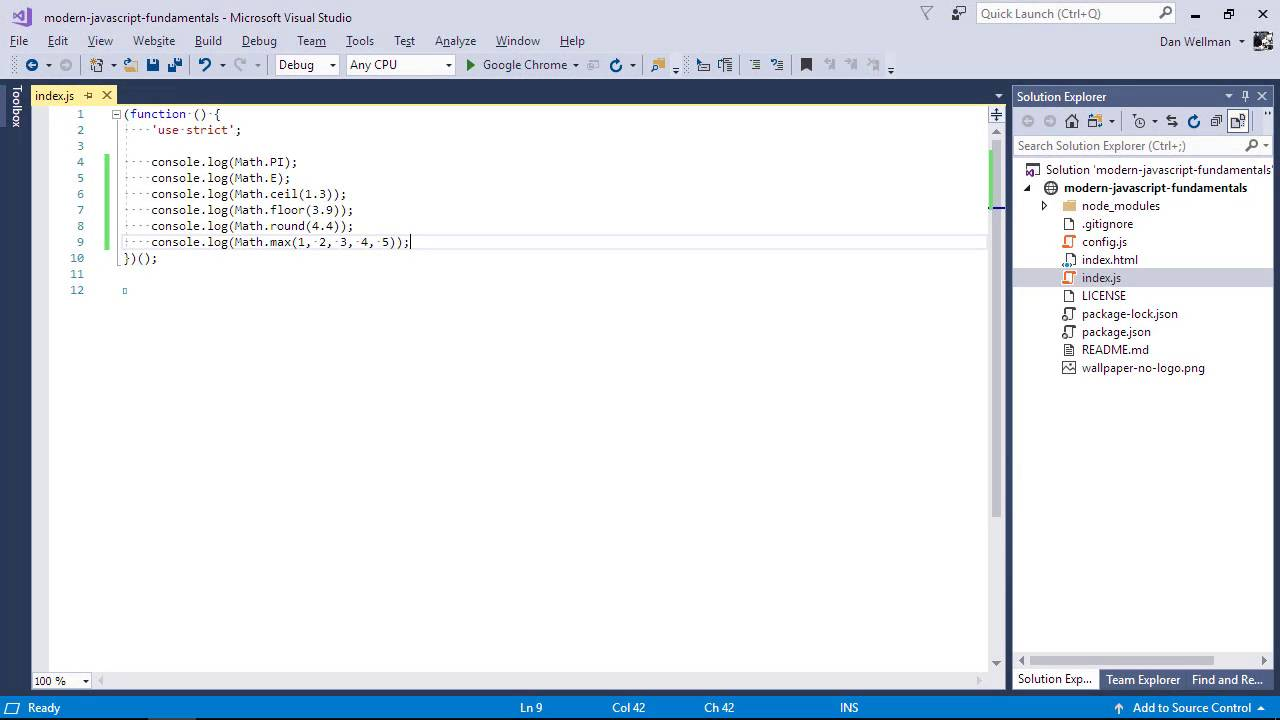Lessons: 65Length: 7.1 hours• Overview
• Transcript

# 5.11 The Math API

In this lesson we’ll take a look at the built-in Math object, which provides properties and methods to perform operations on numbers.

Syntax

• PI
• E
• ceil
• floor
• round
• max
• min
• abs
• sqrt
• pow
• random

## 11.Conclusion1 lesson, 00:43

### 5.11 The Math API

Hi folks. In this lesson, we're going to take a look at the Math object to see how we can use it to perform more advanced mathematical operations than the regular operators like plus or multiply allow. Unlike most other objects in JavaScript, the math object doesn't have a constructor, and it doesn't need to be created. It exists in the browser as a static object which is global, so we can use it anywhere. It has a range of different properties, which are all constant values and cannot be modified. For example, if we want to know the value of Pi, we can use the Pi constant. See how we didn't have to instantiate the math object. It's just there whenever we need it. As well as Pi, we also have constants for Euler's constant. And there's a whole bunch of other things as well like the natural logarithm of 2, and the square root of one half. I didn't study math past high school, so I have no idea what these are used for. But they're there if we need them. The math object also has a range of different methods for working with numbers. Let's look at some of them now. If we want to round a number up to the nearest integer, we can use the ceil method. Or if we want to round a number down to the nearest integer we can use the floor method. So you can see that the rounding with ceil or floor just rounds either up or down. It doesn’t take into account whether the fraction is greater than 0.5 or not. We can also round to the nearest integer using the round method. The round method rounds to the nearest integer. It doesn't just blindly round up or down. So that's rounding covered. What else can we do? We can find the largest of a series of numbers using the max method. So the max method simply returns the largest number out of all of the arguments passed to it. We also have the min method, which does the opposite. We can find the absolute value of a number if we wish, which basically means any positive number. And we do this using the abs method. We can find the square root of any number using the SQRT method. This only works with positive numbers. If we pass the SQRT method a negative number, it returns the null value. We can also raise a number by an exponential power using the power method. So what this does is raise the number 3 by 2 powers. This one of the method that can be pretty useful and that is the random method which allows us to generate a random number between 0 and 1. And if we just refresh that a few times, we should find that the last console log is different each time. So random by itself returns a floating point number in between 0 and 1. If we want to generate a random whole number, we need to floor it and multiply it by 10. And this time, we get a random integer. There are also a range of methods that we can use for trigonometry including cos, sin, and tan. As well as acos, asin, and atan. And future versions of JavaScript will have even more. So in this lesson, we looked at the built-in math object and saw some of the different properties that are available for us to use to perform mathematical operations on numbers. In the next lesson, we're going to see how we can work with dates and times in JavaScript. Thanks for watching.

Back to the top